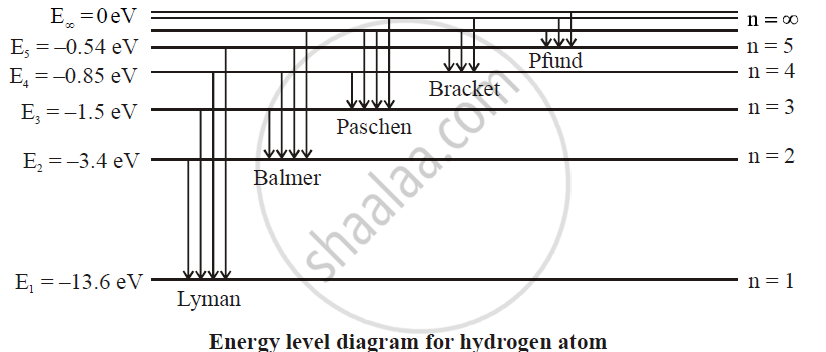# Draw a Neat and Labelled Energy Level Diagram and Explain Balmer Series and Brackett Series of Spectral Lines for Hydrogen Atom. - Physics

Draw a neat and labelled energy level diagram and explain Balmer series and Brackett series of spectral lines for the hydrogen atom.

#### SolutionBalmer series: The spectral lines of this series corresponds to the transition of an electron from some higher energy state to 2nd orbit. For Balmer series, p = 2 and n = 3, 4, 5. The wave numbers and the wavelengths of spectral lines constituting the Balmer series are given by.

barv = 1/lambda  = R(1/2^2 - 1/n^2)

This series lies in the visible region.

Bracket series: The spectral lines of this series corresponds to the transition of an electron from a higher energy state to the 4th orbit.

For this series, p = 4 and n = 5, 6, 7, ....

The wave numbers and the wavelengths of the spectral lines constituting the Bracket series are given by

barv = 1/lambda = R(1/4^2 - 1/n^2)

These series lie in the near infrared region of the spectrum.

Concept: Hydrogen Spectrum
Is there an error in this question or solution?